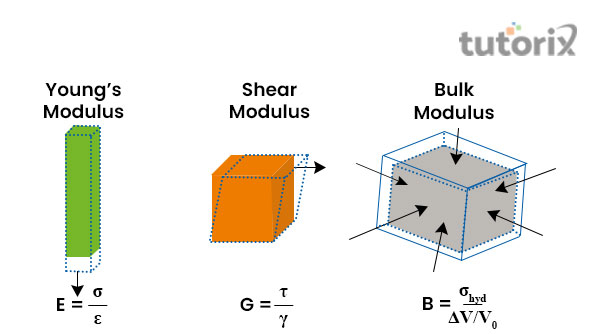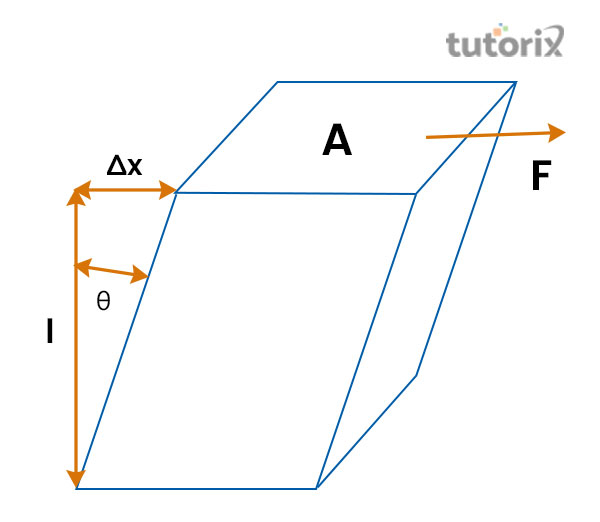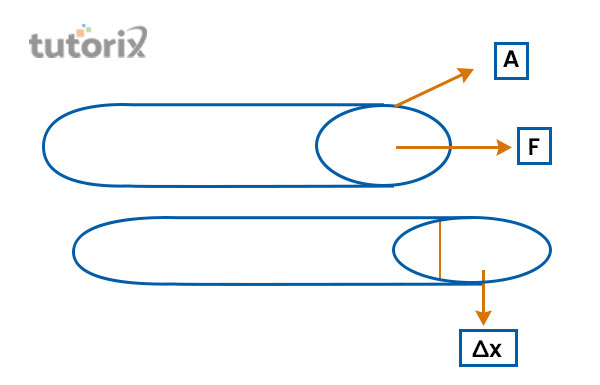# Shear Modulus Elastic Moduli

## Introduction

Shear Modulus of elasticity refers to the mechanical properties of solid objects. Other types of elastic moduli are Young’s modulus and bulk modulus. These particular modules provide a ratio of the shear stress in respect of the sheer strain of a mechanical body. The Pascal or Pa measures the Shear Modulus Elastic Moduli, which is the SI unit of this property. The dimensional formula of the shear modulus refers to M1L-1T-2. Shear modulus is generally represented by G. F denoted the applied force to the objects and Ac stands for the crosssection area of the object perpendicular to the force that has been applied.

## Young’s Modulus of ElasticityFigure 1: Different types of elastic constants

The different types of elastic constants are bulk modulus which is presented by the symbol of K. Young’s modulus or modulus of Elasticity that is denoted by E (Adhikari, Mukhopadhyay & Liu, 2021).

Poisson’s Ratio is specified by µ. Among these types Shear modulus or modulus of rigidity is the most important as this represents the perfect ratio of shear strain and shear stress.

Besides this, this is also very helpful to define the rigidity of any kind of material (Adhikari, Mukhopadhyay & Liu, 2019). For example, the Shear modulus of the wooden particles is 6.2 × 108 Pa while the Shear modulus of steel is 7.2 × 1010 Pa.

## The formula of Shear modulus elastic moduli

The SI unit of the Shear modulus elastic moduli is Pascal which is abbreviated as Pa or N/m2 (Adhikari, Mukhopadhyay & Liu, 2019).

The primary formula of this unit is the diffusion of shear stress and shear strain while the dimension formula is represented as M1L-1T-2Figure 2: Sheer modules

In the above figure, the shear modulus formula is presented in a graphical figure. Here, F stands for the force that is applied to the body and the A denotes the cross-section area of the material perpendicular in terms of the force that has been applied to the body (Wu et al. 2018). In this graphical presentation, Δx denotes the displacement of the transverse.

## The difference between Modulus of Rigidity and Modulus of Elasticity

Modulus of Rigidity Modulus of Elasticity
The modulus of rigidity or the shear modulus is a kind of property of a material whose value is equivalent to the value of the division of shear stress and shear strain (Mukhopadhyay, Adhikari & Alu, 2019). Elasticity is a type of property that mainly explains material deformation. During the exertion of the force of the solid objects, the elasticity undergoes deformation.
It is an elasticity coefficient for the force of elasticity. The deformation is elastic in terms of a smaller deformation like the object returns to its original shape after the forced removal (Wu et al. 2018).
It can be determined by measuring the stress-strain curve slope that is created at the time of the tensile test while conducted on a sample material (Heap et al. 2020). The size of an object is equivalent to the applied force on it and in this case, the Hooke’s law is fully obeyed.
It guides to measuring of the object's deformation while the deforming force is put at the right angle of the surface. It is helpful to measure the object's deformation at the time of applied force to a parallel surface.

Table : A comparison of Modulus of Rigidity and Modulus of Elasticity

## Relation between shear modulus and elastic moduliFigure 3: Shear stress model

Relation between shear modulus and elastic moduli can be presented by the formula 2G(1 + υ) = E = 3K(1 − 2υ) in which G represents the shear modulus, E refers to the Young’s Modulus and K stands for Bulk Modulus. In the formula, u represents the ratio of Poisson (Heap et al. 2020). The value of shear force and shear modulus of an object is equally proportional which means if the sheer force of the object is increased then the rate of shear modulus is equally increased as well.

## Conclusion

Shear modulus is also known as the modules of rigidity as well as shear modulus of elasticity which represents the ratio of a triangular force that is calculated as per unit in terms of the applied area to the machinery body that resulted from the tangential strain within the limitation of elastic. The modulus of elasticity is one of the principal features in the process of calculation in terms of deformation that is produced by the provided stress system that acts on the objects.

## FAQs

Q1. What is the relation between the rigidity modulus to other types of elastic moduli?

Ans: The relation between the rigidity modulus to other types of elastic moduli can be presented with the formula 2G (1 + υ) = E = 3K (1 − 2υ). The relation between shear modulus, Young’s modulus and bulk modulus is easily presented with the ratio of Poisson.

Q2. What happens in case of shear force is increased?

Ans: In the case of improvement of shear force, the rate is also increased with an equivalent proportion of shear modulus. As mentioned, with the reduction of the applied shear force of an object the rate of shear modulus is also decreased.

Q3. Which object is with the highest elasticity level?

Ans: Several metals consist of higher elasticity levels but steel is the material that has the highest elasticity. Examples of dimensionless quantities are the ratio of Poisson and the strain.

Q4. What is the relation between Modulus of Elasticity and Shear Modulus?

Ans: G denotes the modulus of rigidity of an object and the E is the abbreviation form of modulus of elasticity. The relation between these two can be presented with the formula of E = 2G (1 + μ) and the measurement process is based on the Pascal or Pa which is the SI unit of these.